> Example: Create a guided loft with offset scaling

Example: Create a guided loft with offset scaling
Specify an offset value to be modified along the spine.
1. Follow steps 1-5 in Create a surface with Guided Loft.
2. In Variation Options, type a value for the Offset. The offset is a length value applied to the profile. The value can be positive, negative, or zero.
For a specific point on the profile, the offset direction lies in the profile plane and is measured from the point toward the face normal (shiny side) of the surface. A negative offset or a negative scaling leads to an offset to the -face normal (dull side).
3. Modify the offset value with a scaling function that will modulate the offset value along the spine. Select an option from Scale along Spine. These options are the same as those in scalar variation.
With None, the offset value is applied to the entire surface.
If you select the 2D Curve option, you must specify a 2D Curve and Origin.
4. Click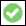to complete the operation.
Reverse - Switch the start and end points of the 2D curve.
Examples
A spine, two profiles, and a workplane with a 2D curve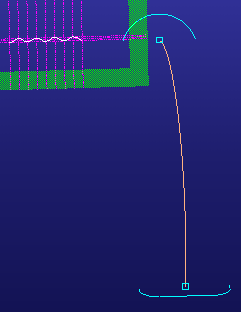Parabolic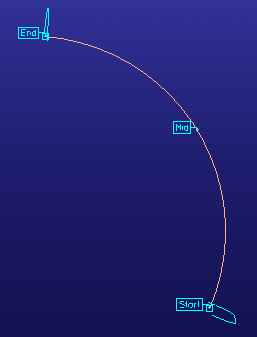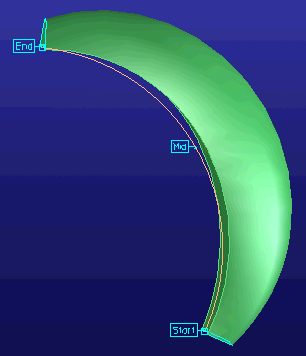The Parabolic option offers a Start, Mid, and End point which will be scaled according to the Offset value. In this example, the Start and End values are 0, the Mid value is 2, and the Offset value is 0.5. The values are added to the profiles at the start, middle, and end position of the spine.
Start = 0.5 mm x 0 = 0 mm
Mid = 0.5 mm x 2 = 1 mm
End = 0.5 mm x 0 = 0 mm
The intermediate values between the start, mid, and end values are calculated by interpolation. The values are approximate.
2D curve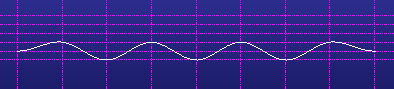A 2D curve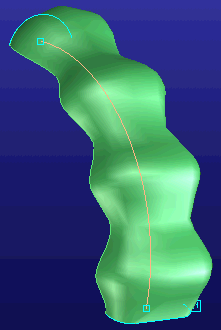The result of the offset scaling for a 2D curve.
Choose an Origin for the 2D curve. In this example, the start point of the 2D curve was chosen as the origin so the scaling function is 0 at the start of the spine. The offset value is then multiplied by the scaling function. The result is 0 and the profiles will not be changed.
Limitations
The offset calculated using Offset is a rough approximation. A precise offset would lead to expensive surfaces.
When Offset is used, birails are not interpolated.